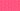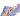PricingResources

# What is Geometric Mean?

Written by

Geometric mean gives the true picture of an investment or portfolio performance by providing an accurate average of investment returns over a long period of time. Keep reading to find out what geometric mean is and how it differs from arithmetic mean.

## Introduction to Geometric Mean

Geometric mean is an average of returns of a group of values, derived by multiplying its terms. The total of multiplied terms is then set to the 1/nth power. For instance, where the values are 3, 3 & 9, the total of multiplied terms is 81. When ‘81’ is set to the 1/nth power, i.e. power of 1/3, the final answer is 3.

Geometric mean is a math tool most notably used in finance. It is capable of producing accurate averages of returns of financial assets over a long period of time. Geometric mean doesn’t require the actual amounts invested, only returns on assets are calculated.

But what makes geometric mean most significant is that it calculates not only the returns on reinvested earnings from an asset, but also the effects. This is called compounding, and the end goal is to give a true picture of investment or portfolio performance using the geometric mean formula.

## Geometric Mean Formula Explained

The geometric mean formula looks like this:

where:

R1....Rn are returns of an asset per period.

Explaining the geometric mean formula:

All series multiplied together are raised to the power of one divided by the total number of series. In calculating financial assets, '1' is typically added to each series to avoid having negative percentages as results. So, this ‘1’ is finally subtracted from the initial answer.

## Geometric Mean Example

The returns of a \$20,000 investment earned from 2010 to 2018 are 13%, 9%, 8%, 11%, 2%, 15%, 4%, 7% and 5%.

Applying the geometric mean formula:

μ geometric =  [( 1 + 13% )( 1 + 9% )( 1 + 8% )(1 + 11%)( 1 + 2% )( 1 + 15% )( 1 + 4% ) (1 + 7% ) (1 + 5% ) ] 1/9  – 1

= (174,182,400%) 1/9 – 1

= 8.24% – 1

= 7. 24%.

The geometric mean is 7.24%

If the above returns were calculated with the arithmetic mean formula, a different answer would be obtained. The relationship between arithmetic mean and geometric mean however goes beyond their different answers only.

## Geometric Mean v. Arithmetic Mean

• First, unlike the geometric mean which is the “nth root product of n numbers”, the arithmetic mean divides the sum of a count by the number of counts.

• The geometric mean is usually lower than the arithmetic mean because it takes compounding into account. But an arithmetic mean is usually higher because it’s calculated as a simple average.

• Unlike the arithmetic mean, geometric mean applies to positive data sets only.

• Extreme numbers have a less significant effect in case of geometric mean, unlike arithmetic mean. For instance, in a number set like 20, 11, 500 and 700, the geometric mean is 93.7, but 307.8 for arithmetic mean.

Geometric mean is ideal for calculating returns of assets typically stretching across a long period of time. This is especially so because the effects of compounding are also accounted for.

## We can help

GoCardless helps you automate payment collection, cutting down on the amount of admin your team needs to deal with when chasing invoices. Find out how GoCardless can help you with ad hoc payments or recurring payments.## Interested in automating the way you get paid? GoCardless can help

Contact sales

Sales

Contact Sales

+1(415) 523-2279

Support

help@gocardless.com

+1 (628) 241-0044